Osam Javed & Kadie Jaffe | CS 184

# Final Project: Fluid Simulation

## Abstract

For our final project, we implemented fluid simulation. Our implementation was based on Position Based Fluids by Macklin and Muller . The approach was to simulate water, an incompressible fluid by enforcing a density constraint on each particle. We used the poly6 and spiky gradient smoothing kernels to distribute our particles throughout the simulation. For each particle we applied a gravitational force, predicted the change in position, and then calculated a correction for the change in position such that our density constraint would be satisfied (and particles wouldn't remain in the same position). This correcting position can be found by applying Newton's method along the constraint gradient. The project required a lot of parameter tuning to ensure that any external force applied to the particles and the pressure force from the smoothing kernel were in an appropriate balance.

## Technical approach

The entire fluid simulation can be implemented via the pseudocode shown below.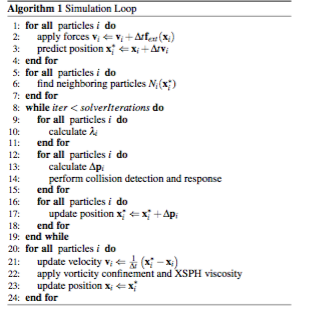</tr> </table> </div>

The accuracy of the fluid simulation relies on the position of each particle, hence the name of the paper 'Position Based Fluids'. We use a particle and it's 'neighboring' particles as input into the smoothing kernel functions we used to distribute our particles and maintain consistent density in our closed system. Water, the fluid we chose to simulate, is incompressible by nature. In order to have our simulation appear accurate, we needed to enforce incompressibility. Each particle relies on surrounding particles to be part of it's neighboring set. The accuracy of the particle's positions and predicted positions was critical to not only the appearance of a frame of the simulation, but also to the next frame since the positions of each particle's neighbors were used to calcuate future positions. Should a particle's neighboring set be empty, we would be calculating the density constraint independent of other particles. For most cases that leads to an unrealistic output, as we wanted all of our particles working together to create a smooth visual simulation.

Initially, we had all of our particles offset from the y-axis so that we could see the effect of gravity as the simulation started. We applied a Vector3D force (0, -9.8, 0) on our particles as the gravitational force. The time step we chose for our simulation was dt = 0.001. The predicted position of each particle would reflect where it's next position would be as a result of it's previous position plus the velocity direction and magnitude. One of the errors we had with gravity was with the way we were calculating our time step. We were using our total time elapsed in the kinematic position equation for gravity, as opposed to one time step. This made our gravitational force too powerful. Once we had a constant change in time, our gravity looked much more realistic. Next, we calculated the scaling factor, which determines the density constraint of a particle given the gradient of all it's neighbors. Initially we had our epsilon value in Equation 11 of the Macklin and Muller paper set to 0.001 which was far too small and caused many errors.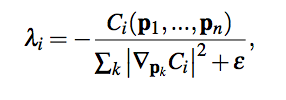We later set this value to 5000. When we realized the effect of gravity was being overshadowed significantly by the particles' interactions with eachother, we increased the epsilon value to a whopping 150,000. Increasing the epsilon value effectively reduced the net force applied by our smoothing kernels. Another challenge we encountered was understanding equation 8, the gradient constraint function.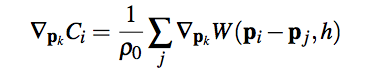We thought the gradient constraint function was multipying the spiky kernel by the poly6 kernel. It turns out that we were supposed to take the gradient of the spiky kernel, which is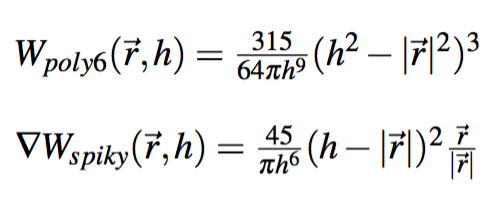</tr> </table> </div>

Once we started using the spiky gradient kernel for calculating our scaling factor and our correcting position update, we got much better results in the density distribution of our particles.

When calculating change in position, we had some particles clustering in the middle of our simulation. We reduced this effect by introducing an artifical pressure term as suggested by Muller. This alleviated the problem of when particles had few neighboring particles since the kernel functions would assume very little density. The premise of the whole paper is finding the correct change in position such that we satisfy our density constraint and have a plausible simulation. It was difficult to debug the change in position since it relied on getting the scaling factor correct and calculating the gradient constraint function accurately. At times we thought we were having issues with our implementation when in reality we just needed to tune our parameters.

As for the collision detection and response, we simply put a particle back inside the boundary of the container if it ended up outside. However, we found a huge bug the night before our presentation. When a particle would hit the floor, the particle's velocity in the y-component would continue to decrease. Resetting this component to zero allowed the smoothing kernels to make more reasonable calculations when determining how much to move a particle. And finally once we have the predicted position and delta position, we can update the particle's actual position.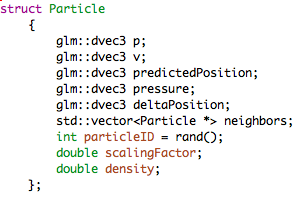</tr> </table> </div>

Debugging this fluid simulation project was an enormous challenge. The problem with fluid simulation projects in general is that they require a lot of parameter tuning. We found that implementing the paper and using the suggested constants did not work for us. We had to fine tune our parameters such that the output simulation looked plausible. In addition, we found ourselves to be wasting a lot of time by quickly changing code and then running the simulation, hoping to get a better result. The reason why this approach doesn't work for us is because we had no clear expectation of what to expect as a result of changing a specific parameter. Towards the end of the project, we found printing the Particle member variables, and adjusting our parameter values to satisfy the density constraint to be zero, led in great improvements.

The key takeaway from the semester was that understand the big picture before jumping into editing code is the most effective and efficient way to work. With graphics projects especially, the more we thought critically about what we were seeing, the more we understood. It is important to be deliberate with changing parameters. We achieved the best results when we changed them one at a time, predicted what we were going to see, and then analyze the result and whether it matched our expectation.

## Results

#### Contributions

Great team effort. Were were responsible in writing the following functions.
Osam Javed
• Particle struct.
• Simulation Loop (outline)
• Collision Detection
• Poly6 Kernel
• Artificial Pressure
• Vector Magnitude
• Neighbor Search
• Particle struct.